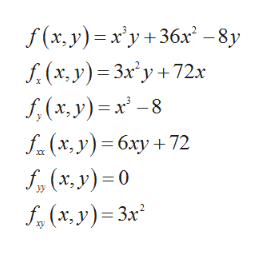Find the local maximum and minimum values and saddle point(s) of the function. If you have three-dimensional graphing software, graph the function with a domain and viewpoint that reveal all the important aspects of the function. (Enter your answers as a comma-separated list. If an answer does not exist, enter DNE.)f(x, y) = x3y + 36x2 − 8y

Question

Find the local maximum and minimum values and saddle point(s) of the function. If you have three-dimensional graphing software, graph the function with a domain and viewpoint that reveal all the important aspects of the function. (Enter your answers as a comma-separated list. If an answer does not exist, enter DNE.)

f(x, y) = x3y + 36x2 − 8y
Step 1

Consider the given function,

Compute the derivative of given function,

Step 2

The derivative of the provided function are as follows,help_outlineImage Transcriptionclosef(x, y) x'y+36x-8y f(x, y) 3xy+72x (x,y)-8 f(x,y)6xy 72 f(x, y)0 (x,y) 3x fullscreen
Step 3

To find the critical points, solve fx(x,y) = 0 and fy(x,y...

Want to see the full answer?

See Solution

Want to see this answer and more?

Our solutions are written by experts, many with advanced degrees, and available 24/7

See Solution
Tagged in

Math